Beyond Seq2Seq with Augmented RNNs by Edward Grefenstette, Google, Inc.

This talk summarizes the limitations of RNN (including LSTM, GRU), from both empirical and computational hierarchy mechanism perspectives.

— Edward Grefenstette
http://videolectures.net/deeplearning2016_grefenstette_augmented_rnn/

Introduction

This talk gives us a general idea of what is the intuition behind the selection of a particular model to solve a particular problem, and what is the process to improve and extend models. This talk summarizes the limitations of RNN (including LSTM, GRU), from both empirical and computational hierarchy mechanism perspectives. The presenter also talks about extending the simple RNN by adding memory which forms a class of memory-based recurrent networks models, including attention model, neural stack model and neural turing machines.

Summary

RNN have been successfully applied to various natural language problems, such as language modeling, sequence labeling, and sequence classification. RNN provides a way to model the conditional likelihood of sequence, which is the fundamental problem of natural language processing.

sequence to sequence mapping with RNN

Many NLP problem tasks are castable as transduction problems:
Translation: English to French transduction
Parsing: String to tree transduction
computation: Take input data, and map input data to the computation results, which is an input data to output data transduction.

Formally speaking, seq2seq model aims to transform some source sequence s (s=s1, s2,…,sm) to some target sequence t (t=t1, t2, …, tn). In order to represent s and model P(t_{i+1} | t_1…t_n;s) with RNNs, we do:

1. Read in source sequence to produce s.
2. Train model to maximize the likelihood of t given s.
3. Test time: generate target sequence t (greedily, beam search, etc) from s.

The bottleneck for simple RNNs(basic, GRU, LSTM):1. Not-adaptive capacity: the hidden representation size is fixed. When decoding, all the information required to produce the English sentence need to be in the fixed size last hidden representation (red unit) of the French encoder . If you have longer and longer sequence in the encoding side, the last hidden unit of the encoder will compress more and more information to a fixed size vector. Therefore, more information will be lost in the compression process.
2. Gradient-starved encoder: in simple RNN, the encoder only get gradients from a single backward pass, which is an imperfect gradient transfer.
3. Target sequence modeling dominates training; in simple RNN, when the decoder generate a English sentence, it tends to ignore the information from the encoder.

Computational limitation of RNNs:

Mathematical theoretical computing mechanisms represent different complexity of computations. A hierarchical diagram of computational mathematical model is shown below:

1. Turing machines: is an abstract machine that manipulates symbols on a strip of tape according to a table of rules. It can represent all computable functions.
2. Pushdown automata: Add stack to FSM, allowing the recognition of context free languages.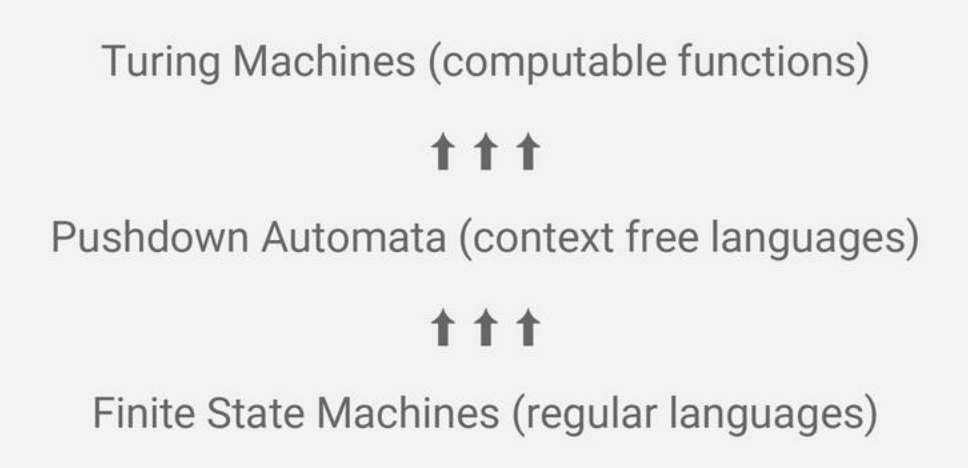3. Finite state machines(FSM): The FSM can change from one state to another, in response to some external inputs. This has an equivalent to regular languages, which can be recognized by regular expression.

According to Sieglemann and Sontag (Sieglemann & Sontag, 1995) , the relationship between RNN and Turing Machines are:

1. Any Turing Machine can be translated into RNN.
2. RNNs can express/approximate a set of Turing Machines. But expressivity is not equal to learnability.
3. Simple RNNs (basic, GRU, LSTM) cannot learn Turning Machines.
1. RNNs cannot control the “tape” in Turing Machines. Sequence exposed in forced order.
2. Maximum likelihood objectives produce model close to training data distribution.
3. Insane to expect regularization to yield structured computational model as an out-of-sample generalization mechanism.

We are expecting that RNN could be a Turing Machine. But currently (from empirical experiences), RNN can only reach Finite State Machines:

1. Effectively order-N Markov Chains, but N need not to be specified.
2. Memoryless in theory. But can simulate memory through dependencies.
3. We are on the FSM state, but we want to be Pushdown Automata and Turing Machines.

RNN revisited:

Consider RNNs as a generic API

1. Keepgradients differentiable, use Previous/Next to track state, do everything you want within the cell.
2. Cells can nest/stack/be sequenced.
3. We can address the problem “RNN still at Finite State Machine state” with this API by separating memory and controller.

The Controller-Memory split

1.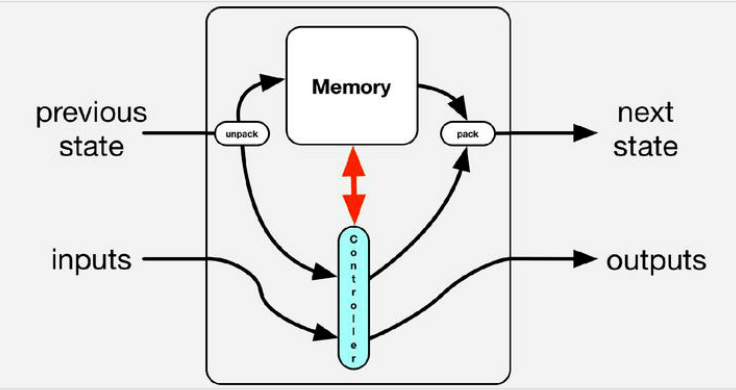Controller could be a RNN, LSTM, GRU which deal with input and output.
2. Controller has interaction with memory.
3. The form of interaction is the main difference of Attention, Stacks

Attention: Read only memory (ROM) fit the RNN API

Attention:

1. You have an array of vectors representing some data.
2. Your controller deals with I/O logic.
3. You want to read your data array to each timestep, and consume the data by either change the internal representation or affect the output of the controller.
4. You want to accumulate gradients in your memory.

How attention model fit the RNN API:

1. Early Fusion: The controller is updated and conditioned on inputs, previous state and some read from memory.2. Late Fusion: Internal state will tell the model which memory to look at, and use that to influence the outputs of controllerROM for encoder-decoder models:

1. Encoder produces array of representations. e.g. one vector per token.
2. Representations are packed into attention matrix.
3. Decoder is a “controller plus memory” model with attention matrix as memory.
4. Gradients of error with respect to memory provide gradients of error with respects to encoder.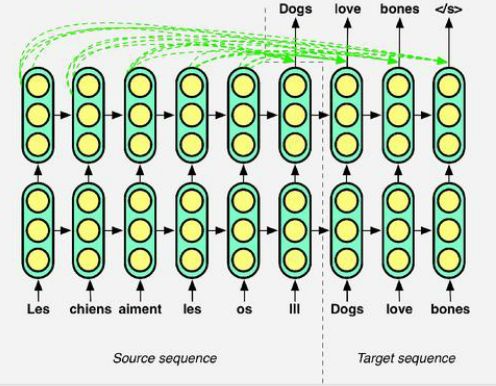1. Encoder is gradient starved no more, it has gradient coming from memory.
2. Compute soft alignments between sequences.
3. Search for information in larger sequences.
4. Memory is not touched, so operations can be done in place.

Applications:

1. Recognizing Textual Entailment (RTE) : Given a pair of sentences, tell whether these two sentences are contradiction/entailment/neutral. 
• Allows you to provide alignment between premise and hypothesis.
• Prediction in hypothesis sentence is not only depends on the final state of premise, but also all words in premise sentence.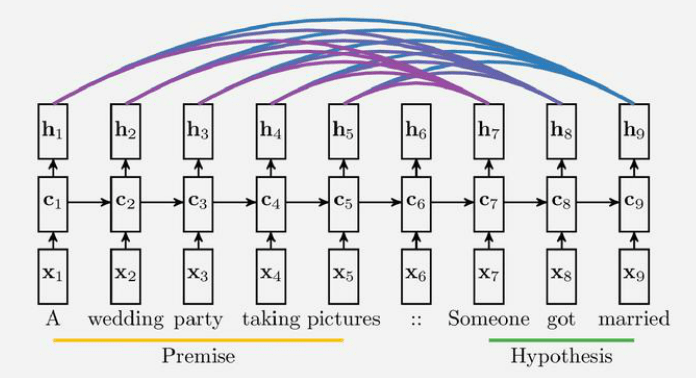2. Machine Reading with attention: 

Stacks: Neural PushDown Automata

Controlling a Neural Stack:During encoding: RNN cell read the input from document, then produce distribution over next symbol/label.
During decoding: RNN cell read the previously generated symbol, then produce distribution over next symbol.
Interaction between RNN cells and a Neural Stack. At each time step, RNN cells write(push) to stack and next time step it will read(pop) from stack.
Neural Stack fits the controller API: Insert a complex differentiable structure into the RNN API.

1. RNNtakes previous read vector r_(t-1) and input i_t. Output the prediction of the symbol (o_t), and the input of neural stack (v_t, u_t, d_t)
2. The neural stack, which is acted upon by a controller by receiving, from the controller, a value v_t, a pop signal u_t∈(0,1), and a push signal d_t∈(0,1). It output a read vector r_t. The neural stack also take pair (v_(t-1), s_(t-1) from previous state, then output the next pair (v_t, s_t). The next pair will use to generate next state H_t.

Not only limited to stack, you can put any differentiable structure into the RNN API, such as Queue and DeQue. The models are being tested with several tasks, such as copy a string and reverse a string. The result shows that adding stack or other structure to LSTM outperforms regular Deep LSTM model. The results are a strong empirical evidence to show that enhance RNN with a data structure which allows to take a step up to the computational hierarchy, is able to learn generalized solution much faster and accurate.Register Machines: Turing Machines (RAM, random access memory)

It uses a similar form of memory mechanism, but with a more sophisticated type of addressing that uses both content-based and location-based addressing, allowing the network to learn the addressing pattern to execute simple computer programs, like sorting algorithms.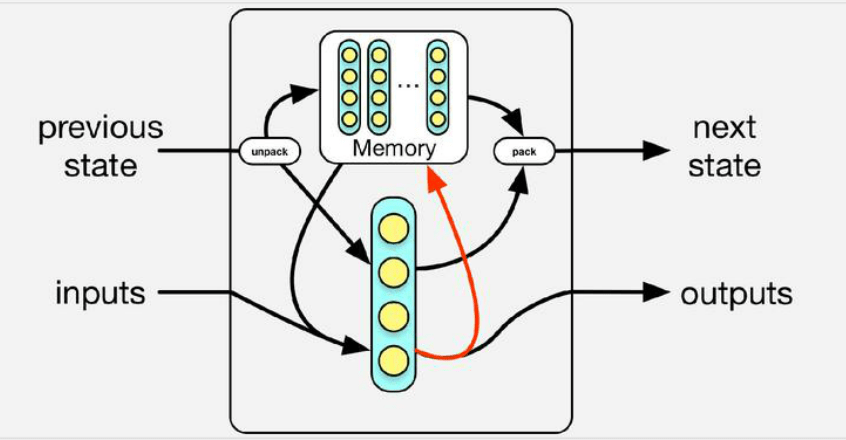1. Read the memory similar to attention.
2. Each time step, the controller will also update the memory.

Extensions:

1. Location based addressing.
2. Mix of location and content-based addressing method.
3. Hard addressing with REINFORCE.
4. More heuristic addressing mechanisms.

Final thoughts:

1. Memory-based RNN enable the network to access internal memory when the model is predicting outputs.
2. In attention model, the attention matrix is not changing when doing prediction, the controller need to learn how to link a word with another word. Therefore, the controller has a lot of pressure to do this task. It is good to alleviate.

Related paper:

learning to execute:https://arxiv.org/pdf/1410.4615.pdf, github:https://github.com/wojciechz/learning_to_execute
On the computational power of Neural Nets(1995): https://binds.cs.umass.edu/papers/1995_Siegelmann_JComSysSci.pdf
 Stanford Natural Language Inference Corpus: http://nlp.stanford.edu/projects/snli/
 Teaching Machine to Read and Comprehend:https://arxiv.org/abs/1506.03340
 Learning to Transduce with Unbounded Memory: https://arxiv.org/pdf/1506.03340.pdf
ATTENTION AND MEMORY IN DEEP LEARNING AND NLP: http://www.wildml.com/2016/01/attention-and-memory-in-deep-learning-and-nlp/

Analyst: Yuting Gui| Localized by Synced Global Team : Xiang Chen